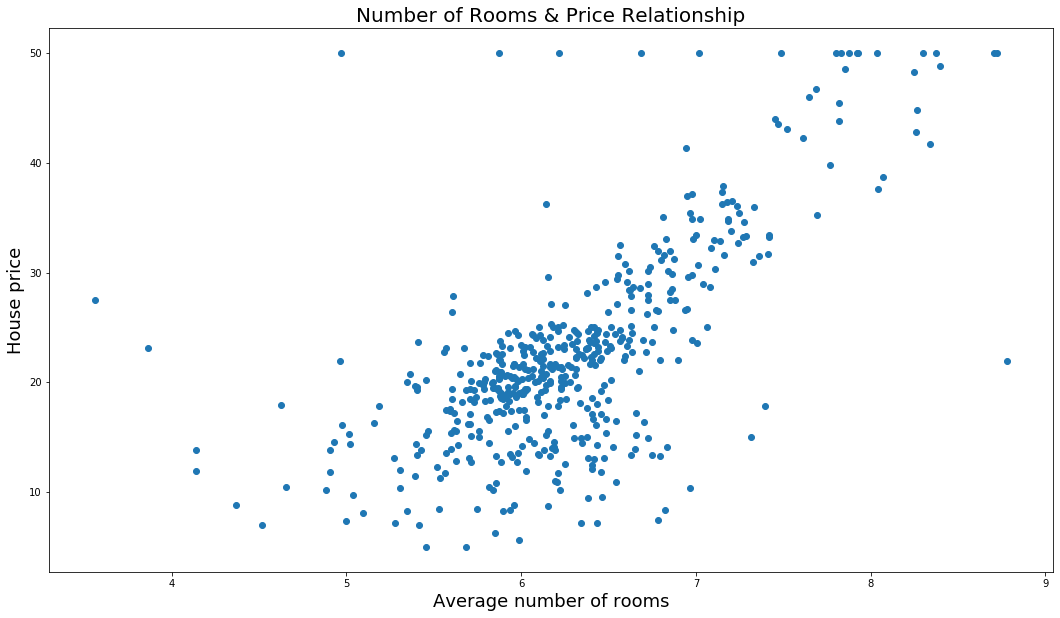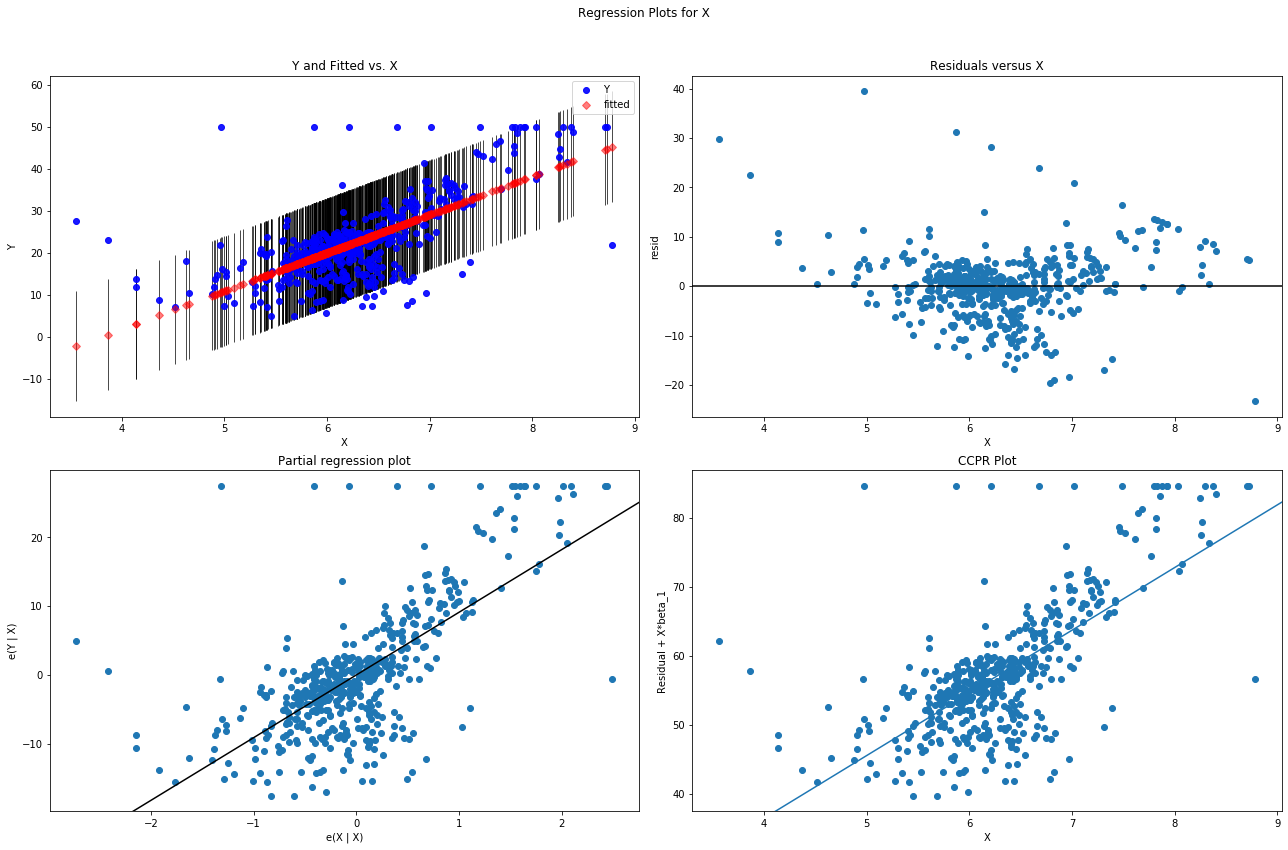## Forecasting – OLS RegressionBoston house prices dataset. This dataset was taken from the StatLib library which is maintained at Carnegie Mellon University.

Data Set Characteristics:
Number of Instances: 506
Number of Attributes: 13 numeric/categorical predictive
Median Value  is usually the target

Data source: Boston Housing Dataset

# Boston housing price forecasting¶

In :
```# Import necessary libraries
import pandas as pd
import scipy.stats as stats
import matplotlib.pyplot as plt
%matplotlib inline
import sklearn
import statsmodels.api as sm
```
In :
```# Importing Boston dataset from sklearn
# Description of Boston house prices dataset
print(boston.DESCR)
```
```.. _boston_dataset:

Boston house prices dataset
---------------------------

**Data Set Characteristics:**

:Number of Instances: 506

:Number of Attributes: 13 numeric/categorical predictive. Median Value (attribute 14) is usually the target.

:Attribute Information (in order):
- CRIM     per capita crime rate by town
- ZN       proportion of residential land zoned for lots over 25,000 sq.ft.
- INDUS    proportion of non-retail business acres per town
- CHAS     Charles River dummy variable (= 1 if tract bounds river; 0 otherwise)
- NOX      nitric oxides concentration (parts per 10 million)
- RM       average number of rooms per dwelling
- AGE      proportion of owner-occupied units built prior to 1940
- DIS      weighted distances to five Boston employment centres
- TAX      full-value property-tax rate per \$10,000
- PTRATIO  pupil-teacher ratio by town
- B        1000(Bk - 0.63)^2 where Bk is the proportion of blacks by town
- LSTAT    % lower status of the population
- MEDV     Median value of owner-occupied homes in \$1000's

:Missing Attribute Values: None

:Creator: Harrison, D. and Rubinfeld, D.L.

This is a copy of UCI ML housing dataset.
https://archive.ics.uci.edu/ml/machine-learning-databases/housing/

This dataset was taken from the StatLib library which is maintained at Carnegie Mellon University.

The Boston house-price data of Harrison, D. and Rubinfeld, D.L. 'Hedonic
prices and the demand for clean air', J. Environ. Economics & Management,
vol.5, 81-102, 1978.   Used in Belsley, Kuh & Welsch, 'Regression diagnostics
...', Wiley, 1980.   N.B. Various transformations are used in the table on
pages 244-261 of the latter.

The Boston house-price data has been used in many machine learning papers that address regression
problems.

.. topic:: References

- Belsley, Kuh & Welsch, 'Regression diagnostics: Identifying Influential Data and Sources of Collinearity', Wiley, 1980. 244-261.
- Quinlan,R. (1993). Combining Instance-Based and Model-Based Learning. In Proceedings on the Tenth International Conference of Machine Learning, 236-243, University of Massachusetts, Amherst. Morgan Kaufmann.

```
In :
```#df = pd.DataFrame(boston.data, columns = boston.feature_names)
```
In :
```# Local file
```
In :
```df.info()
```
```<class 'pandas.core.frame.DataFrame'>
RangeIndex: 506 entries, 0 to 505
Data columns (total 15 columns):
Unnamed: 0    506 non-null int64
CRIM          506 non-null float64
ZN            506 non-null float64
INDUS         506 non-null float64
CHAS          506 non-null float64
NOX           506 non-null float64
RM            506 non-null float64
AGE           506 non-null float64
DIS           506 non-null float64
TAX           506 non-null float64
PTRATIO       506 non-null float64
B             506 non-null float64
LSTAT         506 non-null float64
Price         506 non-null float64
dtypes: float64(14), int64(1)
memory usage: 59.4 KB
```
In :
```df
```
Out:
Unnamed: 0 CRIM ZN INDUS CHAS NOX RM AGE DIS RAD TAX PTRATIO B LSTAT Price
0 0 0.00632 18.0 2.31 0.0 0.538 6.575 65.2 4.0900 1.0 296.0 15.3 396.90 4.98 24.0
1 1 0.02731 0.0 7.07 0.0 0.469 6.421 78.9 4.9671 2.0 242.0 17.8 396.90 9.14 21.6
2 2 0.02729 0.0 7.07 0.0 0.469 7.185 61.1 4.9671 2.0 242.0 17.8 392.83 4.03 34.7
3 3 0.03237 0.0 2.18 0.0 0.458 6.998 45.8 6.0622 3.0 222.0 18.7 394.63 2.94 33.4
4 4 0.06905 0.0 2.18 0.0 0.458 7.147 54.2 6.0622 3.0 222.0 18.7 396.90 5.33 36.2
501 501 0.06263 0.0 11.93 0.0 0.573 6.593 69.1 2.4786 1.0 273.0 21.0 391.99 9.67 22.4
502 502 0.04527 0.0 11.93 0.0 0.573 6.120 76.7 2.2875 1.0 273.0 21.0 396.90 9.08 20.6
503 503 0.06076 0.0 11.93 0.0 0.573 6.976 91.0 2.1675 1.0 273.0 21.0 396.90 5.64 23.9
504 504 0.10959 0.0 11.93 0.0 0.573 6.794 89.3 2.3889 1.0 273.0 21.0 393.45 6.48 22.0
505 505 0.04741 0.0 11.93 0.0 0.573 6.030 80.8 2.5050 1.0 273.0 21.0 396.90 7.88 11.9

506 rows × 15 columns

In :
```# Plotting price dependances from the number of rooms
ax = plt.subplots(figsize=(18,10))
plt.scatter(df.RM, df.Price)
plt.xlabel('Average number of rooms', fontsize=18)
plt.ylabel('House price', fontsize=18)
plt.title ('Number of Rooms & Price Relationship', fontsize=20);
```In :
```# Declaring X as average number of rooms per dwelling
X = df[['RM']]
```
In :
```# Declaring Y as target
Y = df[['Price']]
```
In :
```from sklearn.linear_model import LinearRegression
lm = LinearRegression()
lm
```
Out:
`LinearRegression(copy_X=True, fit_intercept=True, n_jobs=None, normalize=False)`
In :
```# Fitting the data
lm.fit(X, Y)
```
Out:
`LinearRegression(copy_X=True, fit_intercept=True, n_jobs=None, normalize=False)`
In :
```print('Estimated intercept coefficient:', lm.intercept_)
print('Number of coefficients:', lm.coef_)
```
```Estimated intercept coefficient: [-34.67062078]
Number of coefficients: [[9.10210898]]
```
In :
```# Linear regression plot
fig, ax = plt.subplots(figsize=(18,10))
plt.scatter(X, Y)
plt.plot(X, lm.predict(X), 'red')
plt.xlabel('Average number of rooms', fontsize=18)
plt.ylabel('House price', fontsize=18)
plt.title ('Number of Rooms vs. Price Relationship Regression', fontsize=20);
```In :
```# Import stats models to get regression results
from statsmodels.formula.api import ols
```
In :
```# Regression results
m = ols('Y ~ X', df).fit()
print(m.summary())
```
```                            OLS Regression Results
==============================================================================
Dep. Variable:                      Y   R-squared:                       0.484
Method:                 Least Squares   F-statistic:                     471.8
Date:                Fri, 25 Oct 2019   Prob (F-statistic):           2.49e-74
Time:                        13:33:42   Log-Likelihood:                -1673.1
No. Observations:                 506   AIC:                             3350.
Df Residuals:                     504   BIC:                             3359.
Df Model:                           1
Covariance Type:            nonrobust
==============================================================================
coef    std err          t      P>|t|      [0.025      0.975]
------------------------------------------------------------------------------
Intercept    -34.6706      2.650    -13.084      0.000     -39.877     -29.465
X              9.1021      0.419     21.722      0.000       8.279       9.925
==============================================================================
Omnibus:                      102.585   Durbin-Watson:                   0.684
Prob(Omnibus):                  0.000   Jarque-Bera (JB):              612.449
Skew:                           0.726   Prob(JB):                    1.02e-133
Kurtosis:                       8.190   Cond. No.                         58.4
==============================================================================

Warnings:
 Standard Errors assume that the covariance matrix of the errors is correctly specified.
```
In :
```# Regression exploration plots
fig = plt.figure(figsize=(18,12))
fig = sm.graphics.plot_regress_exog(m, 'X', fig=fig)
```